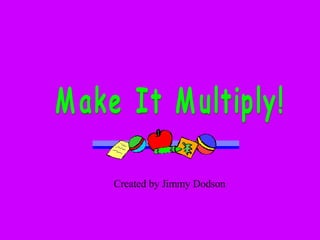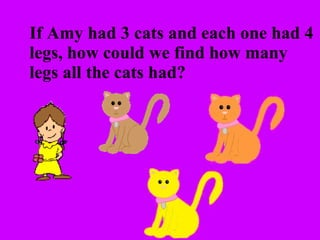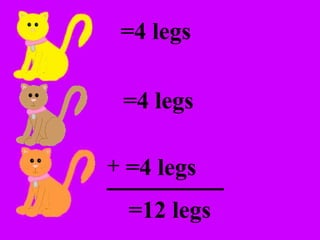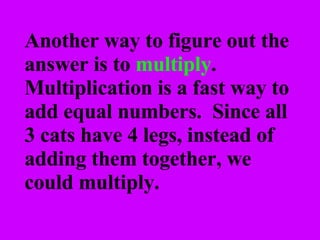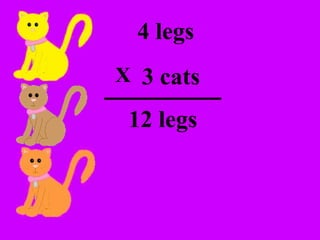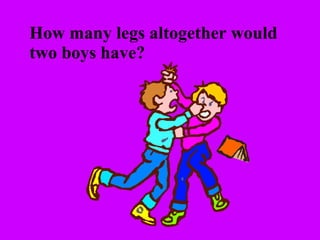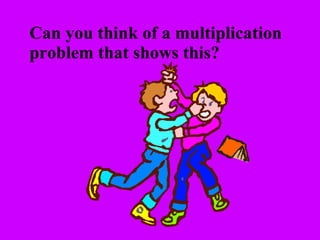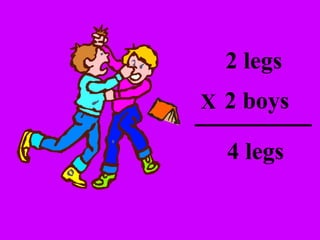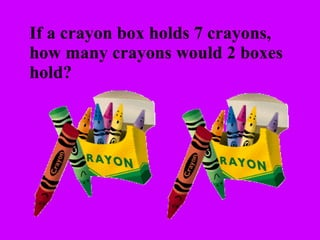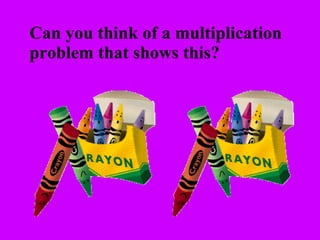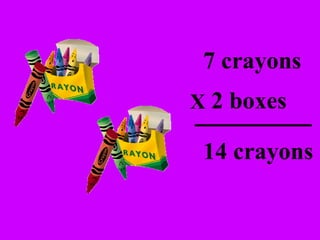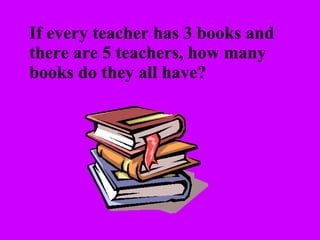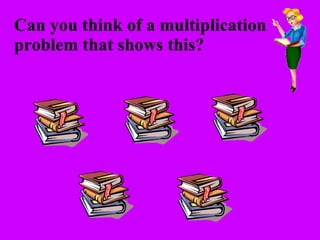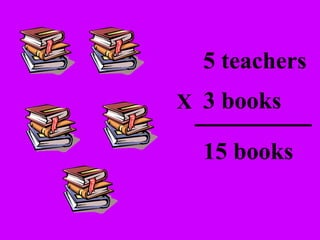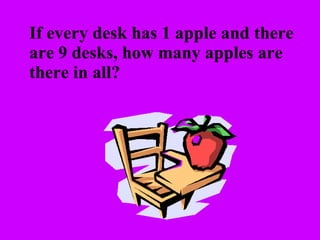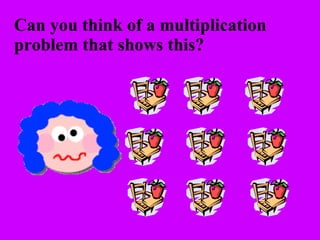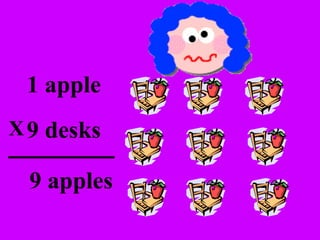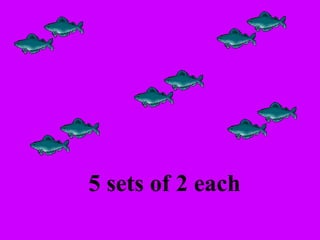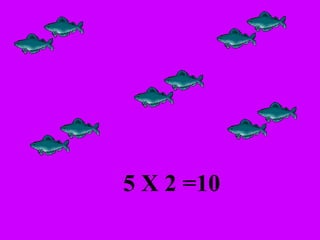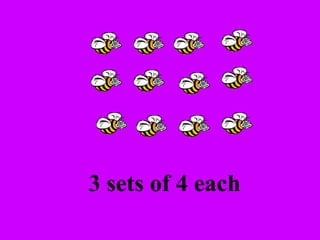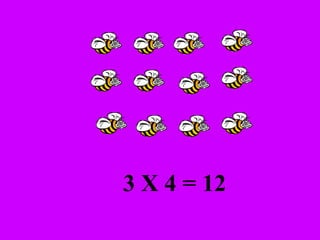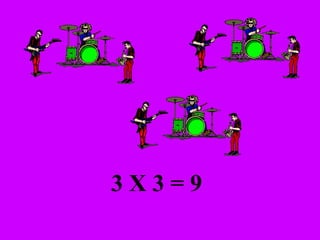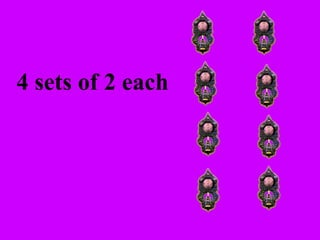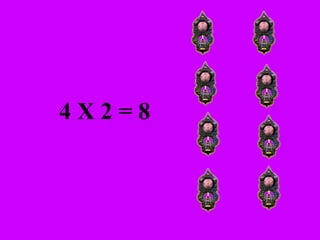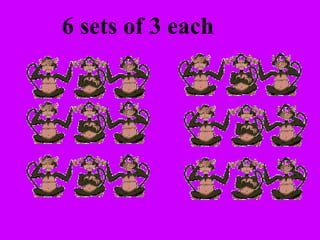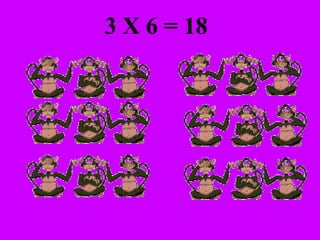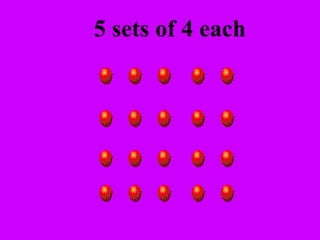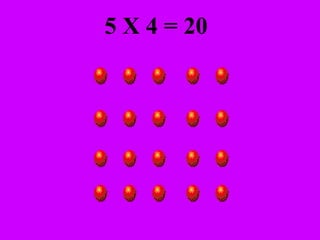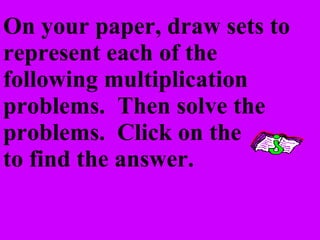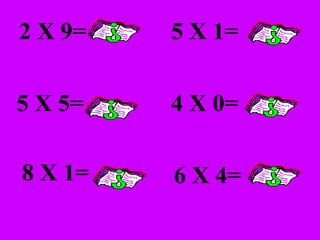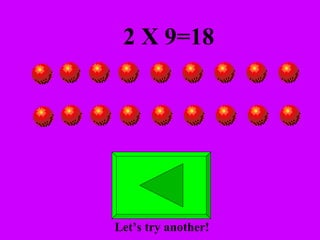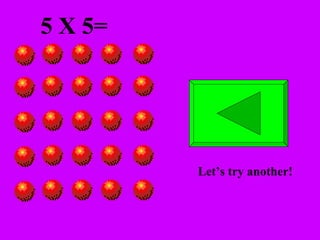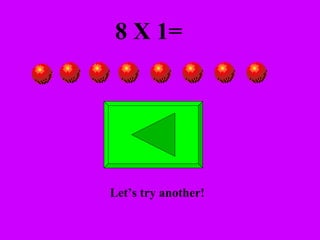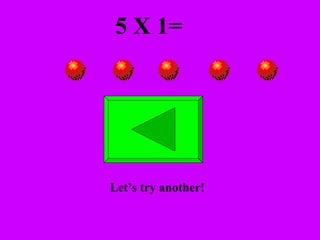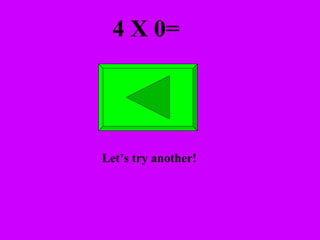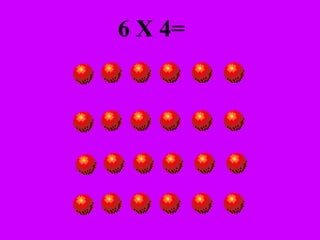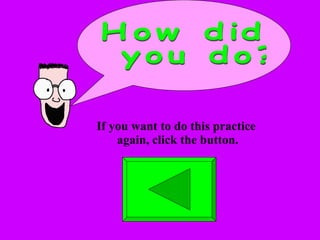1 of 39

### Multiplication Powerpoint

1. Make It Multiply! Created by Jimmy Dodson
2. If Amy had 3 cats and each one had 4 legs, how could we find how many legs all the cats had?
3. =4 legs =4 legs =4 legs =12 legs +
4. Another way to figure out the answer is to multiply . Multiplication is a fast way to add equal numbers. Since all 3 cats have 4 legs, instead of adding them together, we could multiply.
5. 4 legs 3 cats 12 legs X
6. How many legs altogether would two boys have?
7. Can you think of a multiplication problem that shows this?
8. 2 boys 2 legs 4 legs X
9. If a crayon box holds 7 crayons, how many crayons would 2 boxes hold?
10. Can you think of a multiplication problem that shows this?
11. 2 boxes 7 crayons 14 crayons X
12. If every teacher has 3 books and there are 5 teachers, how many books do they all have?
13. Can you think of a multiplication problem that shows this?
14. 3 books 5 teachers 15 books X
15. If every desk has 1 apple and there are 9 desks, how many apples are there in all?
16. Can you think of a multiplication problem that shows this?
17. 1 apple 9 desks 9 apples X
18. Multiplication involves sets of objects. Can you write a multiplcation problem for these sets? I think I can do it!
19. 5 sets of 2 each
20. 5 X 2 =10
21. 3 sets of 4 each
22. 3 X 4 = 12
23. 3 sets of 3 each
24. 3 X 3 = 9
25. 4 sets of 2 each
26. 4 X 2 = 8
27. 6 sets of 3 each
28. 3 X 6 = 18
29. 5 sets of 4 each
30. 5 X 4 = 20
31. On your paper, draw sets to represent each of the following multiplication problems. Then solve the problems. Click on the to find the answer.
32. 2 X 9= 5 X 5= 8 X 1= 5 X 1= 4 X 0= 6 X 4=
33. 2 X 9=18 Let’s try another!
34. 5 X 5= Let’s try another!
35. 8 X 1= Let’s try another!
36. 5 X 1= Let’s try another!
37. 4 X 0= Let’s try another!
38. 6 X 4=
39. How did you do? If you want to do this practice again, click the button.